﻿ 暴雨极值分析 Extreme Value Analysis of Rainstorm

Climate Change Research Letters
Vol. 08  No. 02 ( 2019 ), Article ID: 29068 , 8 pages
10.12677/CCRL.2019.82018

Extreme Value Analysis of Rainstorm

Wenpeng Zhao, Yonglai Zheng*, Yubao Zhou

College of Civil Engineering, Tongji University, Shanghai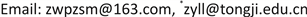Received: Feb. 8th, 2019; accepted: Feb. 21st, 2019; published: Feb. 28th, 2019ABSTRACT

In the context of environmental processes, non-stationarity of precipitation is often apparent because of seasonal effects like monsoon, typhoon, etc. Because non-stationarity violates the assumption of extreme models, this paper adopts the seasonal models that divide the rainfall data into four seasons and are examined by likelihood test. GEV is employed to analyze the monthly maximum rainfall. In addition, degree of experience is used to estimate the maximum return period, that is, how large the extrapolation limit is. The result shows that based on the 90-month rainfall data, the summer rainfall is subject to the Gumbel distribution with an inaccurate extrapolation, while the maximum extrapolation periods in spring, autumn and winter are 54.2, 68.6 and 84.6 months respectively. Therefore, it is recommended that degree of experience could be applied to engineering because of its reliability and practicability.

Keywords:Extreme Analysis, Seasonal Models, Degree of Experience, Return Period, Confidence IntervalCopyright © 2019 by author(s) and Hans Publishers Inc.

This work is licensed under the Creative Commons Attribution International License (CC BY).

http://creativecommons.org/licenses/by/4.0/1. 引言

2. 研究方法

2.1. 泊松分布与极值分布

${F}_{n}\left(y\right)={p}_{n}\left(0\right)=\frac{{\left\{{\lambda }_{n}\left(y\right)\right\}}^{0}}{0!}{\text{e}}^{-{\lambda }_{n}\left(y\right)}$ (1)

${\lambda }_{n}\left(y\right)=\left\{\begin{array}{cc}-{\left(1+\xi \frac{y-{\mu }_{n}}{{\sigma }_{n}}\right)}^{-\frac{1}{\xi }}& \xi \ne 0\\ \mathrm{exp}\left(-\frac{y-{\mu }_{n}}{{\sigma }_{n}}\right)& \xi =0\end{array}$ (2)

${H}_{u}\left(y\right)=\frac{\lambda \left(y,{\theta }_{N}\right)}{\lambda \left(u,{\theta }_{N}\right)}=\begin{array}{cc}\frac{\lambda \left(y,{\theta }_{1}\right)}{\lambda \left(u,{\theta }_{1}\right)}& y>u\end{array}$ (3)

2.2. Delta估计

$\stackrel{^}{\varphi }\sim N\left(\varphi ,{V}_{\varphi }\right)$ (4)

${V}_{\varphi }=\nabla {\varphi }^{T}{V}_{\left(\mu ,\sigma ,\xi \right)}\nabla \varphi$ (5)

$\nabla \varphi ={\left[\frac{\partial \varphi }{\partial \mu },\frac{\partial \varphi }{\partial \sigma },\frac{\partial \varphi }{\partial \xi }\right]}^{T}$ (6)

${V}_{\left(\mu ,\sigma ,\xi \right)}$$\left(\mu ,\sigma ,\xi \right)$ 的方差协方差矩阵，计算时均以估计值代入，此方法即为delta法。

2.3. 季度模型

${Z}_{t}\sim \text{GEV}\left(\mu \left(t\right),\sigma \left(t\right),\xi \left(t\right)\right)$ (7)

${Z}_{t}\sim \text{GEV}\left(\mu \left(t\right),\sigma ,\xi \right)$ (8)

2.4. 似然比检验

2.5. 模型检测

$\stackrel{˜}{{Z}_{t}}=\frac{1}{\stackrel{^}{\xi }\left(t\right)}\mathrm{log}\left\{1+\stackrel{^}{\xi }\left(t\right)\left(\frac{{Z}_{t}-\stackrel{^}{\mu }\left(t\right)}{\stackrel{^}{\sigma }\left(t\right)}\right)\right\}$ (9)

$\left\{i/\left(m+1\right),\mathrm{exp}\left(-\mathrm{exp}\left(-{\stackrel{˜}{z}}_{\left(i\right)}\right)\right);i=1,\cdots ,m\right\}$ (10)

$\left\{{\stackrel{˜}{z}}_{\left(i\right)},-\mathrm{log}\left(-\mathrm{log}\left(i/\left(m+1\right)\right)\right);i=1,\cdots ,m\right\}$ (11)

2.6. 经验度试算

$K=\frac{{E}^{2}\left(\lambda \right)}{V\left(\lambda \right)}$ (12)

3. 实例应用

3.1. 模型拟合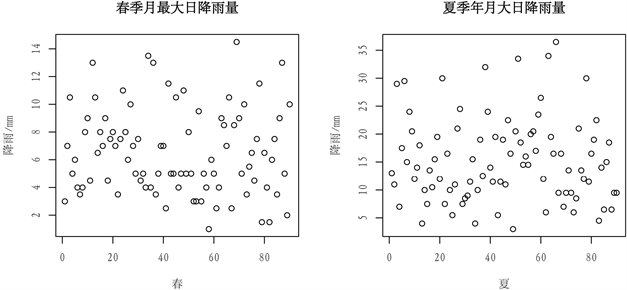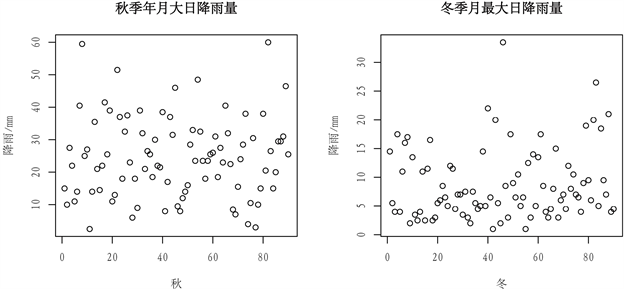Figure 1. Maximum daily rainfall of every month in different seasons

${\mu }_{春}={\mu }_{冬}$ (9)

${\mu }_{夏}={\mu }_{秋}$ (10)Table 1. Estimation of seasonal model parameters

$2\left(-1227.37-\left(-1273.54\right)\right)>qchisq\left(0.95,2\right)$ (11)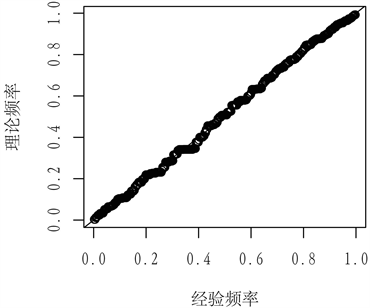(a) 概率图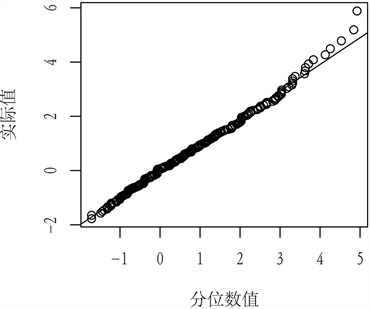(b) 分位数图

Figure 2. Model check plot

3.2. 经验度计算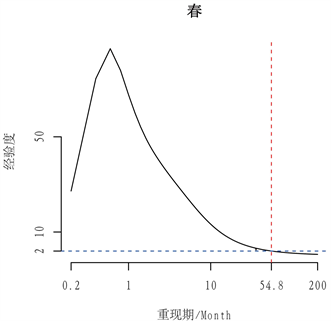(a)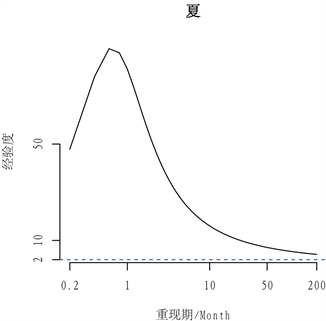(b)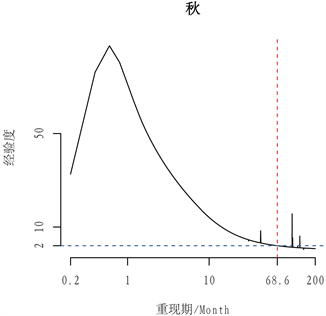(c)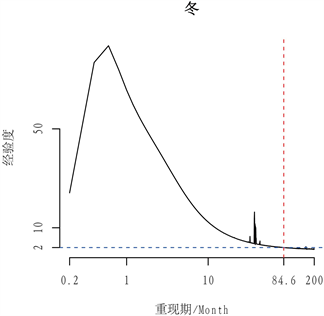(d)

Figure 3. Degree of experience curve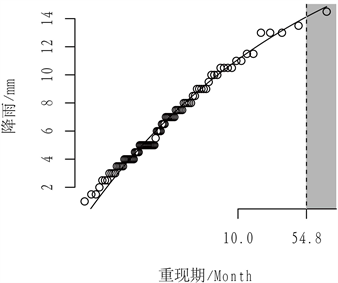(a) 春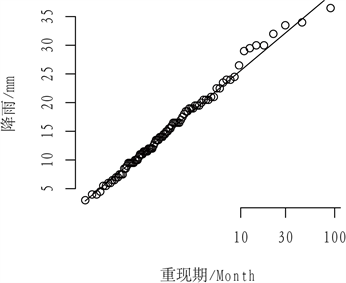(b) 夏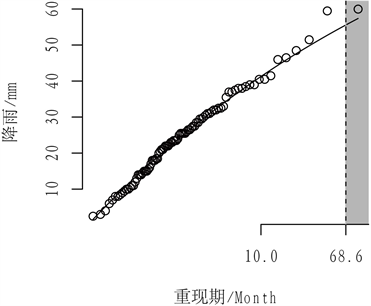(c) 秋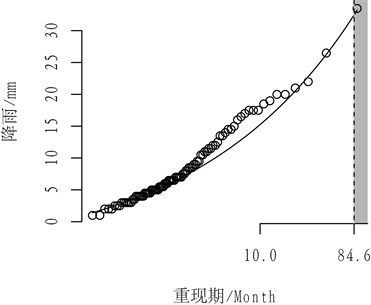(d) 冬

Figure 4. Return level curve

4. 结论

Extreme Value Analysis of Rainstorm[J]. 气候变化研究快报, 2019, 08(02): 160-167. https://doi.org/10.12677/CCRL.2019.82018

1. 1. Coles, S. (2001) An Introduction to Statistical Modeling of Extreme Values. Springer, London. https://doi.org/10.1007/978-1-4471-3675-0

2. 2. IPCC (2018) An IPCC Special Report on the Impacts of Global Warming of 1.5℃ above Pre-Industrial Levels and Related Global Greenhouse Gas Emission Pathways, in the Context of Strengthening the Global Re-sponse to the Threat of Climate Change, Sustainable Development, and Efforts to Eradicate Poverty.

3. 3. 黄维, 杨春友, 张和喜, 等. 贵州省极端气候时空演变分析[J]. 人民长江, 2017(s1): 115-120+165.

4. 4. 张金锋, 刘联兵, 冯雄波. 浅谈极端气候条件下长江流域水资源配置[J]. 人民长江, 2011, 42(18): 91-94.

5. 5. 陈争杰. 极值统计模型在大渡河流域暴雨频率分析中的应用[D]: [硕士学位论文]. 徐州: 中国矿业大学, 2015.

6. 6. 陈子燊, 刘曾美, 路剑飞. 基于广义Pareto分布的洪水频率分析[J]. 水力发电学报, 2013, 32(2): 68-73, 83.

7. 7. Kitano, T., Morise, T., Kioka, W., et al. (2008) Degree of Experience in Statistical Analysis for Extreme Wave Heights. Journal of Japan Society of Civil Engineers, 55, 141-145. https://doi.org/10.2208/proce1989.55.141

8. 8. Kitano, T. and Kioka, W. (2015) Balancing Two Types Errors Arised in Detecting the Difference between the Occurrence Rates of Sea Extremes Due to Climate Change. Journal of Japan Civil Engineers, 71, 97-102.

9. 9. Japan Meteorological Agency (2018) Tropical Cyclone Information. https://www.jma.go.jp/jma/jma-eng/jma-center/rsmc-hp-pub-eg/RSMC_HP.htm

10. NOTES

*通讯作者。